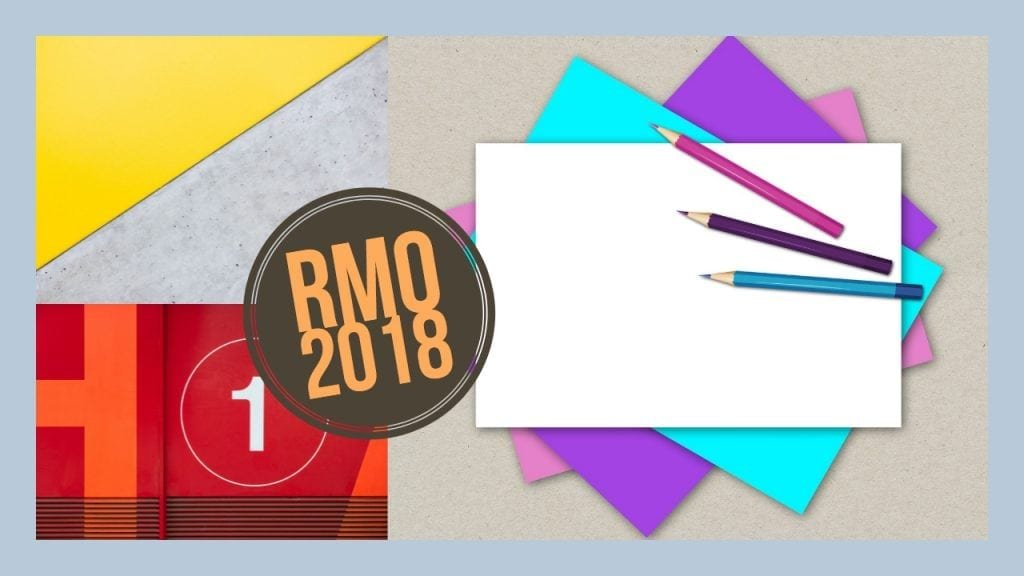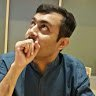How Cheenta works to ensure student success?
Explore the Back-Story

# RMO 2018 Problems, SolutionsThis post contains RMO 2018 solutions, problems, and discussions.

RMO 2018, Problem 1:

Let $ABC$ be a triangle with integer sides in which $AB < AC$. Let the tangent to the circumcircle of triangle $ABC$ at $A$ intersect the line $BC$ at $D$. Suppose $AD$ is also an integer. Prove that gcd$(AB,AC) >1$.

RMO 2018, Problem 2:

Let $n$ be a natural number. Find all real numbers $x$ satisfying the equation    $\displaystyle\sum_{k=1}^{n} \frac{kx^k}{1+x^{2k}}$$=\frac{n(n+1)}{4}$

RMO 2018, Problem 3:

For a rational number $r$, its period is the length of the smallest repeating block in its decimal expansion. For example, the number $r=0.123123123..$ has period $3$. If $S$ denotes the set of all rational numbers $r$ of the form $r=0.\overline{abcdefgh}$ having period $8$, find the sum of all elements of $S$.

RMO 2018, Problem 4:

Let $E$ denotes the set of $25$ points $(m,n)$ in the$xy$-plane, where $m$,$n$ are natural numbers, $1 \leq m \leq 5$, $1 \leq m \leq 5$. Suppose the points of $E$ are arbitrarily coloured using two colours, red and blue. Show that there always  exist  four points in the set $E$ of the form $(a,b)$, $(a+k,b)$, $(a+k,b+k)$, $(a,b+k)$ for some positive integer $k$ such that at least three of these four points have the same colour. ( That is there always  exist  four points in the set $E$ which form the vertices of a square with sides parallel to axes and having at least three points of the same colour.)

RMO 2018, Problem 5:

Find all natural numbers $n$ such that $1+[\sqrt{2n}]$ divides $2n$. (For any real number $x$, $[x]$ denotes the largest integer not exceeding x.)

RMO 2018, Problem 6:

Let $ABC$ be an acute-angled triangle with $AB<AC$. Let $I$ be the incentre of triangle $ABC$, and let $D$,$E$,$F$ be the points at which its incircle touches the side $BC$,$CA$,$AB$ respectively. Let $BI$, $CI$ meet the line $EF$ at $Y$,$X$, respectively. Further assume that both $X$ and $Y$ are outside the triangle $ABC$. Prove that

$B$,$C$,$Y$,$X$ are concyclic; and

$I$ is also the incentre of triangle $DYX$.

## RMO 2018 Tamil Nadu Problems and Solution

1. Let ABC be an acute-angled triangle and let D be an interior point of the line segment BC. Let the circumcircle of triangle ACD intersect AB at E (E between A and B) and let the circumcircle of triangle ABD intersect AC at F (F between A and C). Let O be the circumcenter triangle AEF. Prove that OD bisects ∠EDF
2. Find the set of all real values of $\mathbf{a}$ for which the real polynomial equation $P(x)=x^{2}-2 a x+b=0$ has real roots, given that $P(0) \cdot P(1) \cdot P(2) \neq 0$ and $P(0), P(1), P(2)$ form a geometric progression.

\begin{aligned}&\text { 3.Show that there are infinitely many } 4 \text { -tuples }(a, b, c, d) \text { of natural numbers such that }\&a^{3}+b^{4}+c^{5}=d^{7}
\end{aligned}

Sequential Hints and Discussion

4.Suppose 100 points in the plane are colored using two colors, red and white, such that each red point is the center of a circle passing through at least three white points. What is the least possible number of white points?

5.In a cyclic quadrilateral ABCD with circumcenter O, the diagonals AC and BD intersect at X. Let the circumcircles of triangles AXD and BXC intersect at Y. Let the circumcircles of triangle AXB and CXD intersect at Z. If O lies inside ABCD and if the points O, X, Y, Z are all distinct, prove that O, X, Y, Z lie in a circle.

6. Define a sequence $\displaystyle <a_n>_{n\geq 1}$ of real numbers by

$$a_1=2, a_{n+1}=\frac{a_n^2+1}{2}\text{ for } n \geq 1$$

Prove that

$$\displaystyle\sum_{j=1}^N \frac{1}{a_j+1}<1$$

for every natural number $N$ .

This post contains RMO 2018 solutions, problems, and discussions.

RMO 2018, Problem 1:

Let $ABC$ be a triangle with integer sides in which $AB < AC$. Let the tangent to the circumcircle of triangle $ABC$ at $A$ intersect the line $BC$ at $D$. Suppose $AD$ is also an integer. Prove that gcd$(AB,AC) >1$.

RMO 2018, Problem 2:

Let $n$ be a natural number. Find all real numbers $x$ satisfying the equation    $\displaystyle\sum_{k=1}^{n} \frac{kx^k}{1+x^{2k}}$$=\frac{n(n+1)}{4}$

RMO 2018, Problem 3:

For a rational number $r$, its period is the length of the smallest repeating block in its decimal expansion. For example, the number $r=0.123123123..$ has period $3$. If $S$ denotes the set of all rational numbers $r$ of the form $r=0.\overline{abcdefgh}$ having period $8$, find the sum of all elements of $S$.

RMO 2018, Problem 4:

Let $E$ denotes the set of $25$ points $(m,n)$ in the$xy$-plane, where $m$,$n$ are natural numbers, $1 \leq m \leq 5$, $1 \leq m \leq 5$. Suppose the points of $E$ are arbitrarily coloured using two colours, red and blue. Show that there always  exist  four points in the set $E$ of the form $(a,b)$, $(a+k,b)$, $(a+k,b+k)$, $(a,b+k)$ for some positive integer $k$ such that at least three of these four points have the same colour. ( That is there always  exist  four points in the set $E$ which form the vertices of a square with sides parallel to axes and having at least three points of the same colour.)

RMO 2018, Problem 5:

Find all natural numbers $n$ such that $1+[\sqrt{2n}]$ divides $2n$. (For any real number $x$, $[x]$ denotes the largest integer not exceeding x.)

RMO 2018, Problem 6:

Let $ABC$ be an acute-angled triangle with $AB<AC$. Let $I$ be the incentre of triangle $ABC$, and let $D$,$E$,$F$ be the points at which its incircle touches the side $BC$,$CA$,$AB$ respectively. Let $BI$, $CI$ meet the line $EF$ at $Y$,$X$, respectively. Further assume that both $X$ and $Y$ are outside the triangle $ABC$. Prove that

$B$,$C$,$Y$,$X$ are concyclic; and

$I$ is also the incentre of triangle $DYX$.

## RMO 2018 Tamil Nadu Problems and Solution

1. Let ABC be an acute-angled triangle and let D be an interior point of the line segment BC. Let the circumcircle of triangle ACD intersect AB at E (E between A and B) and let the circumcircle of triangle ABD intersect AC at F (F between A and C). Let O be the circumcenter triangle AEF. Prove that OD bisects ∠EDF
2. Find the set of all real values of $\mathbf{a}$ for which the real polynomial equation $P(x)=x^{2}-2 a x+b=0$ has real roots, given that $P(0) \cdot P(1) \cdot P(2) \neq 0$ and $P(0), P(1), P(2)$ form a geometric progression.

\begin{aligned}&\text { 3.Show that there are infinitely many } 4 \text { -tuples }(a, b, c, d) \text { of natural numbers such that }\&a^{3}+b^{4}+c^{5}=d^{7}
\end{aligned}

Sequential Hints and Discussion

4.Suppose 100 points in the plane are colored using two colors, red and white, such that each red point is the center of a circle passing through at least three white points. What is the least possible number of white points?

5.In a cyclic quadrilateral ABCD with circumcenter O, the diagonals AC and BD intersect at X. Let the circumcircles of triangles AXD and BXC intersect at Y. Let the circumcircles of triangle AXB and CXD intersect at Z. If O lies inside ABCD and if the points O, X, Y, Z are all distinct, prove that O, X, Y, Z lie in a circle.

6. Define a sequence $\displaystyle <a_n>_{n\geq 1}$ of real numbers by

$$a_1=2, a_{n+1}=\frac{a_n^2+1}{2}\text{ for } n \geq 1$$

Prove that

$$\displaystyle\sum_{j=1}^N \frac{1}{a_j+1}<1$$

for every natural number $N$ .

This site uses Akismet to reduce spam. Learn how your comment data is processed.

### 5 comments on “RMO 2018 Problems, Solutions”

1.Ekaveera says:

Hi sir is this question paper of today that is 7th October 2018

1.Ashani Dasgupta says:

Yes

2.Abhishek says:

Problem 2 follows by a simple AM-GM inequality. Upper bound the given quantity Q by replacing x by absolute value of (x), then divide the Numerator and Denominator by |x| to obtain

$Q\leq \sum_{k=1}^{n}\frac{k}{|x|^k+|x|^{-k}}.$
Using the AM-GM inequality, we have
$Q\leq \frac{1}{2}\sum_{k=1}^{n}k=\frac{n(n+1)}{4},$
hence the equality must hold throughout and we have the only solution $x=1$.

3.komal says:

In problem number 4 why the diagonal is RBRBR it can be RRBRR or any other combination . We just don't want 3 consecutive monochromatic

1.Ashani Dasgupta says:

watch Part 2

### Knowledge Partner